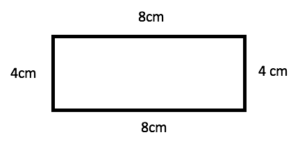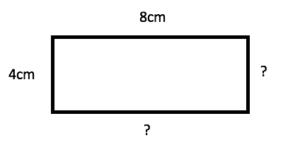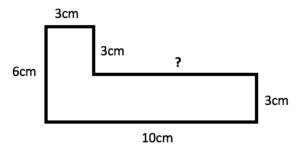## What is the perimeter? The perimeter is the total distance around a two-dimensional (2D) shape.

To find the perimeter of a shape, you just need to add up the lengths of all its sides.

For example:The perimeter of this rectangle 8+4+8+4=24cm.

The perimeter of a circle is called the circumference.

## When do children first learn to calculate the perimeter?

Children will first be taught about the perimeter in Year 4.

Often a useful way of introducing the perimeter is to ask children to think about walking around the sides of a shape as if it were a park. This makes it easier to visualise that the perimeter is the total distance that they have walked.

Teachers may then give children examples like the shape above, and ask them to add up all the measurements to find the perimeter.

## How might children struggle with the perimeter?

Calculating the perimeter can get more confusing when children are given an example where the shape has missing lengths.This requires children to learn that the short sides of a rectangle are the same length and that the long sides are also the same length.  Once they understand this, there are a few ways children can calculate the perimeter of the rectangle:

4+8+4+8, (4+8) x 2, or (4 x 2) + (8 x 2), all giving the answer 24cm.

## More complicated examples

From Year 5 onwards, teachers will give children more complicated shapes with missing lengths and ask them to calculate the perimeter. For example:To work out the missing length of this shape, children must learn to use the other lengths which have been given.

The missing length of this shape must be the length of the bottom line (10), minus the top length of the shape (3): 10-3=7cm.

Eventually, children will be given questions on the perimeter which involve having to visualise the shape for themselves, rather than being given a picture of it. This is important as it encourages children to draw a sketch of the shape themselves, helping them gain confidence in calculating the perimeter.

## How does Learning Street help children with the perimeter?

Learning Street introduces the topic of the perimeter at an appropriate time and then gradually develops a child’s understanding of it. Revision is built in and eventually, children will develop their knowledge sufficiently to perform well in tests.

Tests could include SATs, competitive 11 Plus tests or selective Independent school exams.

## Our Courses

Click through to review the courses we have available

##### Get Started

Learning Street structured courses make home study easier and more successful. There's no need to wonder which books to buy or what to do next. Everything is planned for you.

• Proven to deliver excellent results
• Used by families, private tutors and schools
• Fully planned and structured